# AP Physics C: Mechanics : Circular and Rotational Motion

## Example Questions

← Previous 1 3 4

### Example Question #1 : Circular Motion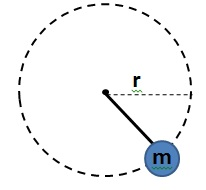A ball of massis tied to a rope and moves along a horizontal circular path of radiusas shown in the diagram (view from above). The maximum tension the rope can stand before breaking is given by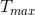. Which of the following represents the ball's linear velocity given that the rope does not break?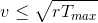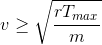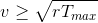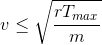None of theseExplanation:

This is a centripetal force problem. In this case the tension on the rope is the centripetal force that keeps the ball moving on a circle.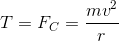If we want for the rope not to break, then the tension should never exceed.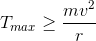Now we just solve for velocity:### Example Question #1 : Rotational Motion And Torque

What is the rotational equivalent of mass?

Moment of inertia

Angular momentum

Torque

Moment of inertia

Explanation:

The correct answer is moment of inertia. For linear equations, mass is what resists force and causes lower linear accelerations. Similarly, in rotational equations, moment of inertia resists torque and causes lower angular accelerations.

### Example Question #41 : Mechanics Exam

In rotational kinematics equations, what quantity is analogous to force in linear kinematics equations?

Torque

Moment of inertia

Impulse

Angular acceleration

Torque

Explanation:

Just as force causes linear acceleration, torque causes angular acceleration. This can be seen most in the linear-rotational comparison of Newton's second law: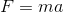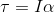### Example Question #49 : Mechanics Exam

A boot is put in a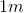stick which is attached to a rotor. The rotor turns with an angular velocity of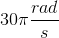. What is the linear velocity of the boot?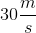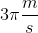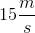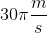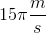Explanation:

Linear (tangential) velocity,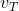is given by the following equation: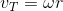Here,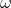is the angular velocity in radians per second andis the radius in meters.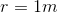Solve.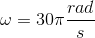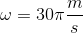### Example Question #1 : Rotational Motion And Torque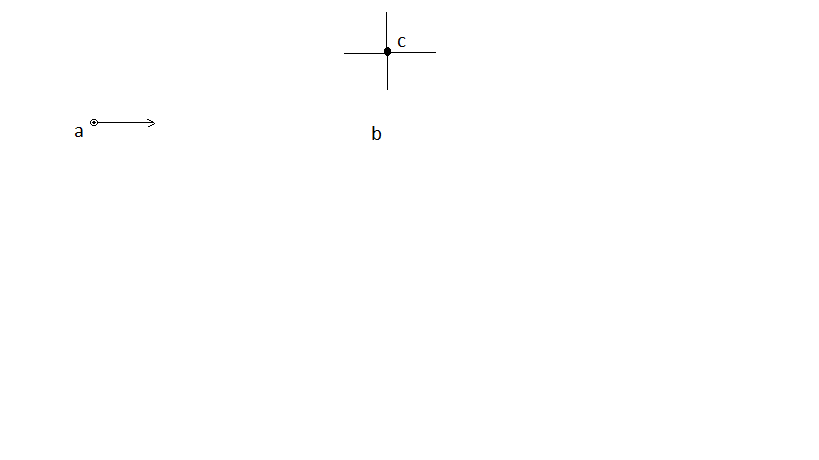A particle is moving at constant speed in a straight line past a fixed point in space, c. How does the angular momentum of the particle about the fixed point in space change as the particle moves from point a to point b?

It cannot be determined without knowing the mass of the particle

The angular momentum increases

The particle does not have angular momentum since it is not rotating

The angular momentum decreases

The angular momentum does not change

The angular momentum does not change

Explanation:

The angular momentum of a particle about a fixed axis is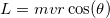. As the particle draws nearer the fixed axis, bothand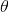change. However, the product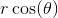remains constant. If you imagine a triangle connecting the three points, the productrepresents the"of closest approach", labeled "" in the diagram.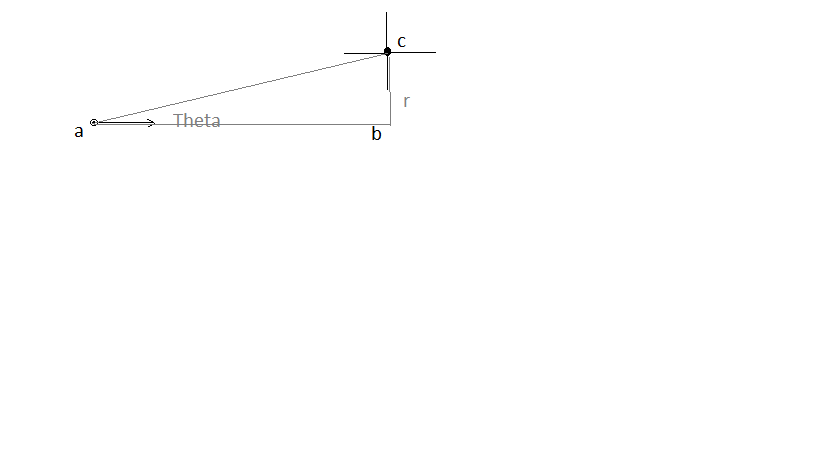### Example Question #2 : Rotational Motion And Torque

Doing which of the following would allow you to find the center of mass of an object?

Spinning it

Hanging it from a fixed point

Sliding it along a flat surface

Recording its shape

Spinning it

Explanation:

Center of mass can be found by spinning an object. It will naturally spin around its center of mass, due to the concept of even distribution of mass in relation to the center of mass. Shape and mass are important factors in this property, but the most improtant factor is the mass distribution.

### Example Question #3 : Circular And Rotational Motion

If the fulcrum of a balanced scale is shifted to the left, what type of adjustment must be made to rebalance the scale?

Apply more mass to the left end

Apply more mass to the right end

Apply more mass to the new position of the fulcrum

Apply the same amount of mass to both ends

If the scale was initially balanced, moving the fulcrum will not change this

Apply more mass to the left end

Explanation:

Changing the position of the fulcrum by moving it to the left means the center of mass will be to the right of the new position. Therefore, the scale will tip right. Adding more mass to the left end will rebalance the scale. None of the other options make sense. Adding more mass to the new fulcrum position will not change the balance of the scale because that mass is a negligible distance from the new fulcrum position and does nothing to change the masses on either side.

### Example Question #4 : Rotational Motion And Torque

If two masses,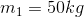and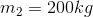are placed on a seesaw of length, where must the fulcrum be placed such that the seesaw remains level?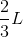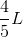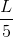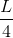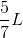Explanation:

This question asks us to find the center of mass for this system. We know that the center of mass resides a distance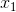from the first mass such that: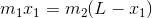In this case: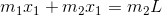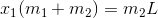Plug in known values and solve.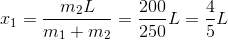### Example Question #5 : Rotational Motion And Torque

Three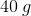point masses are at the points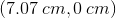,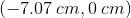and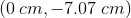and a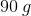point mass is at the point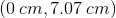.

How far from the origin is the center of mass of the system?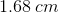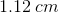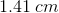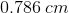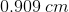Explanation:

To find the center of mass, we have to take the weighted average of the x coordinates and the y coordinates.Measures:MeasuresFirst, we take the weighted measurement of the x-axis: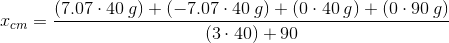We can see that the result of the x-axis contribution is equal to.

Now, let's look at the y-axis contribution: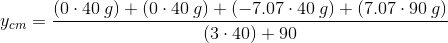This equals toNow that we have the x and y components, we take the root of squares to get the final answer: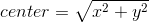This will give us### Example Question #1 : Using Torque Equations

An object starts from rest and accelerates to an angular velocity ofin three seconds under a constant torque of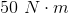. How many revolutions has the object made in this time?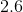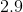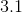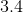Explanation:

Since it is experiencing a constant torque and constant angular acceleration, the angular displacement can be calculated using: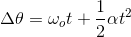The angular acceleration is easily calculated using the angular velocity and the time: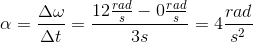Using this value, we can find the angular displacement: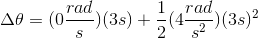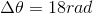Convert the angular displacement to revolutions by diving by: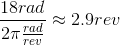← Previous 1 3 4